# VBA SUMIF and SUMIFS Functions

This tutorial will show you how to use the Excel SUMIF and SUMIFS Functions in VBA.

VBA does not have an equivalent of the SUMIF or SUMIFS Functions that you can use – a user has to use the built-in Excel functions in VBA using the WorksheetFunction object.

## SUMIF WorksheetFunction

The WorksheetFunction object can be used to call most of the Excel functions that are available within the Insert Function dialog box in Excel. The SUMIF function is one of them.

``````Sub TestSumIf()
Range("D10") = Application.WorksheetFunction.SumIf(Range("C2:C9"), 150, Range("D2:D9"))
End Sub``````

The procedure above will only add up the cells in Range(D2:D9) if the corresponding cell in column C = 150.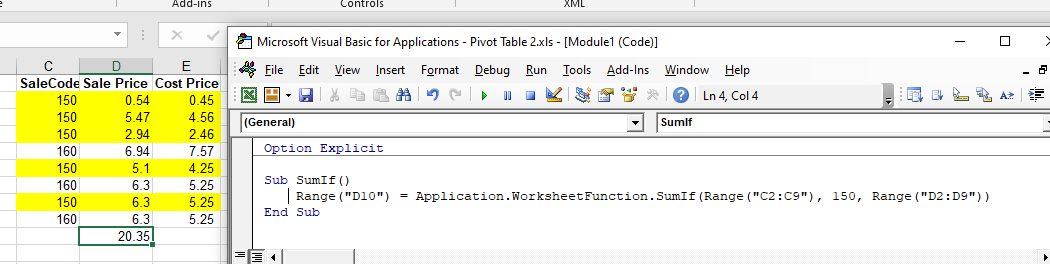## Assigning a SUMIF result to a Variable

You may want to use the result of your formula elsewhere in code rather than writing it directly back to an Excel Range. If this is the case, you can assign the result to a variable to use later in your code.

``````Sub AssignSumIfVariable()
Dim result as Double
'Assign the variable
result = WorksheetFunction.SumIf(Range("C2:C9"), 150, Range("D2:D9"))
'Show the result
MsgBox "The total of the result matching the 150 sales code is " &  result
End Sub``````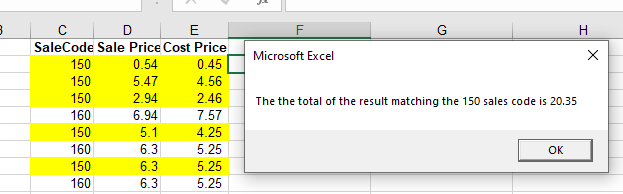## Using SUMIFS

The SUMIFS function is similar to the SUMIF WorksheetFunction but it enables you to check for more than one criteria. In the example below, we are looking to add up the sale price if the sale code is 150 AND the Cost Price is greater than 2. Notice that in this formula, the range of cells to add up is in front of the criteria, whereas in the SUMIF function, it is behind.

``````Sub MultipleSumIfs()
Range("D10") = WorksheetFunction.SumIfs(Range("D2:D9"), Range("C2:C9"), 150, Range("E2:E9"), ">2")
End Sub``````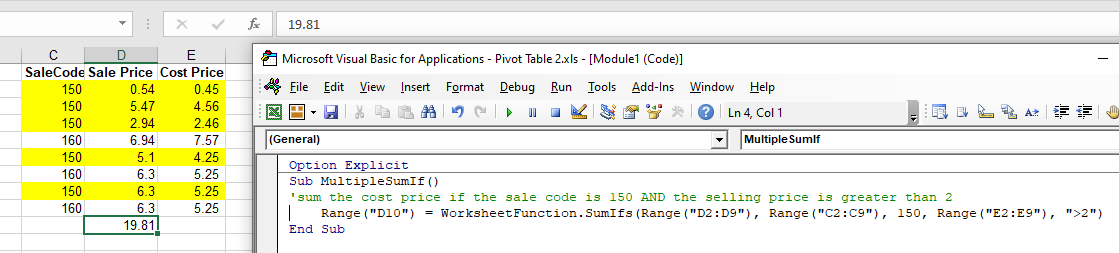## Using SUMIF with a Range Object

You can assign a group of cells to the Range object, and then use that Range object with the WorksheetFunction object.

``````Sub TestSumIFRange()
Dim rngCriteria As Range
Dim rngSum as Range
'assign the range of cells
Set rngCriteria = Range("C2:C9")
Set rngSum = Range("D2:D9")
'use the range in the  formula
Range("D10") = WorksheetFunction.SumIf(rngCriteria, 150, rngSum)
'release the range objects
Set rngCriteria = Nothing
Set rngSum = Nothing
End Sub``````

## Using SUMIFS on Multiple Range Objects

Similarly, you can use SUMIFS on multiple Range Objects.

``````Sub TestSumMultipleRanges()
Dim rngCriteria1 As Range
Dim rngCriteria2 as Range
Dim rngSum as Range
'assign the range of cells
Set rngCriteria1= Range("C2:C9")
Set rngCriteria2 = Range("E2:E9")
Set rngSum = Range("D2:D9")
'use the ranges in the formula
Range("D10") = WorksheetFunction.SumIfs(rngSum, rngCriteria1, 150, rngCriteria2, ">2")
'release the range object
Set rngCriteria1 = Nothing
Set rngCriteria2 = Nothing
Set rngSum = Nothing
End Sub``````

Notice that because you are using a greater than sign, the criteria greater than 2 needs to be within quotes.

## SUMIF Formula

When you use the WorksheetFunction.SUMIF to add a sum to a range in your worksheet, a static sum is returned, not a flexible formula. This means that when your figures in Excel change, the value that has been returned by the WorksheetFunction will not change.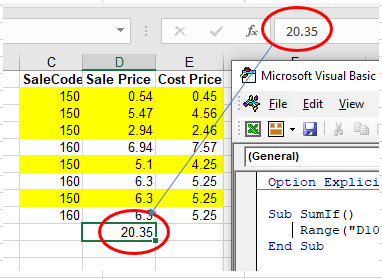In the example above, the procedure has added up Range(D2:D9) where the SaleCode equals 150 in column C, and the result was put in D10. As you can see in the formula bar, this result is a figure and not a formula.

If any of the values change in either Range(D2:D9) or Range(C2:D9), the result in D10 will NOT change.

Instead of using the WorksheetFunction.SumIf, you can use VBA to apply a SUMIF Function to a cell using the Formula or FormulaR1C1 methods.

Stop searching for VBA code online. Learn more about AutoMacro - A VBA Code Builder that allows beginners to code procedures from scratch with minimal coding knowledge and with many time-saving features for all users!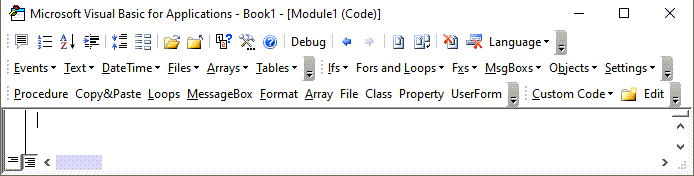### Formula Method

The formula method allows you to point specifically to a range of cells eg: D2:D10 as shown below.

``````Sub TestSumIf()
Range("D10").Formula = "=SUMIF(C2:C9,150,D2:D9)"
End Sub``````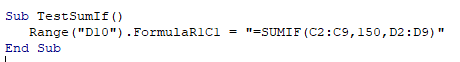### FormulaR1C1 Method

The FormulaR1C1 method is more flexible in that it does not restrict you to a set range of cells. The example below will give us the same answer as the one above.

``````Sub TestSumIf()
Range("D10").FormulaR1C1 = "=SUMIF(R[-8]C[-1]:R[-1]C[-1],150,R[-8]C:R[-1]C)"
End Sub``````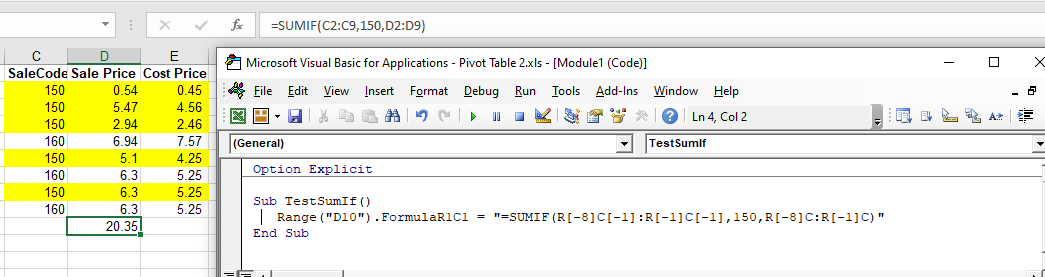However, to make the formula more flexible, we could amend the code to look like this:

``````Sub TestSumIf()
ActiveCell.FormulaR1C1 = "=SUMIF(R[-8]C[-1]:R[-1]C[-1],150,R[-8]C:R[-1]C)"
End Sub``````

Wherever you are in your worksheet, the formula will then add up the cells that meet the criteria directly above it and place the answer into your ActiveCell. The Range inside the SUMIF function has to be referred to using the Row (R) and Column (C) syntax.

Both these methods enable you to use Dynamic Excel formulas within VBA.

There will now be a formula in D10 instead of a value.